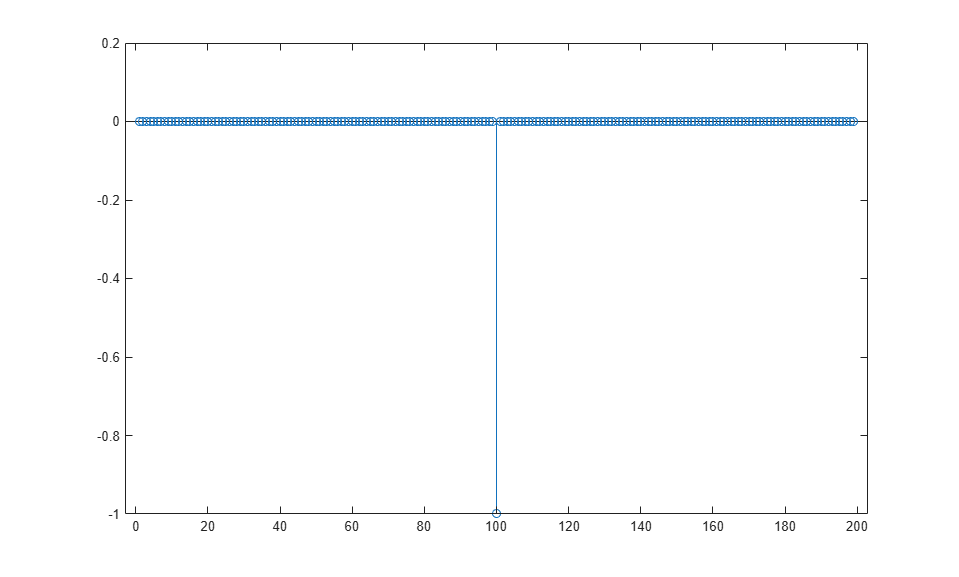firpr2chfb

Two-channel FIR filter bank for perfect reconstruction

Syntax

[h0,h1,g0,g1] = firpr2chfb(n,fp)
[h0,h1,g0,g1] = firpr2chfb(n,dev,'dev')
[h0,h1,g0,g1] = firpr2chfb('minorder',fp,dev)

Description

[h0,h1,g0,g1] = firpr2chfb(n,fp) designs four FIR filters for the analysis sections (h0 and h1) and synthesis section is (g0 and g1) of a two-channel perfect reconstruction filter bank. The design corresponds to the orthogonal filter banks also known as power-symmetric filter banks.

n is the order of all four filters. It must be an odd integer. fp is the passband-edge for the lowpass filters h0 and g0. The passband-edge argument fp must be less than 0.5. h1 and g1 are highpass filters with the passband-edge given by (1-fp).

[h0,h1,g0,g1] = firpr2chfb(n,dev,'dev') designs the four filters such that the maximum stopband ripple of h0 is given by the scalar dev. Specify dev in linear units, not decibels. The stopband-ripple of h1 is also be given by dev, while the maximum stopband-ripple for both g0 and g1 is (2*dev).

[h0,h1,g0,g1] = firpr2chfb('minorder',fp,dev) designs the four filters such that h0 meets the passband-edge specification fp and the stopband-ripple dev using minimum order filters to meet the specification.

Examples

collapse all

Design a filter bank with filters of order n equal to 99 and passband edges of 0.45 and 0.55.

n = 99;
[h0,h1,g0,g1] = firpr2chfb(n,.45);
fvtool(h0,1,h1,1,g0,1,g1,1);Here are the filters, showing clearly the passband edges.

Use the following stem plots to verify perfect reconstruction using the filter bank created by firpr2chfb.

stem(1/2*conv(g0,h0)+1/2*conv(g1,h1))
n=0:n;
stem(1/2*conv((-1).^n.*h0,g0)+1/2*conv((-1).^n.*h1,g1))
stem(1/2*conv((-1).^n.*g0,h0)+1/2*conv((-1).^n.*g1,h1))
stem(1/2*conv((-1).^n.*g0,(-1).^n.*h0)+...
1/2*conv((-1).^n.*g1,(-1).^n.*h1))
stem(conv((-1).^n.*h1,h0)-conv((-1).^n.*h0,h1))Extended Capabilities